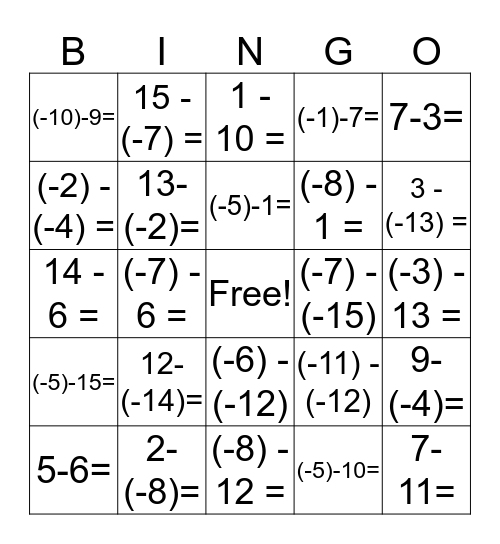# Subtracting Integers BingoThis bingo card has a free space and 59 words: (-15) - 14 =, 2-(-8)=, 11 - (-11) =, (-5)-15=, (-5)-10=, (-9) - (-1) =, 13-(-2)=, 12-(-4)=, (-8) - 1 =, (-8) - (-12), (-1)-7=, 2-(-11)=, (-2) - (-4) =, (-8)-7=, (-8) - (-4) =, (-10)-9=, 7-11=, 7-3=, (-13)-1=, (-5)-1=, (-7) - (-15), 1 - 10 =, 5-6=, 9-(-4)=, 14 - 9 =, 1-6=, (-6)-14=, (-14) - 3 =, 3 - (-13) =, 1 - (-2) =, (-3) - 4 =, 7 - (-3) =, (-13)-12=, (-12) - (-9), 15 - (-7) =, (-15)-15=, (-8) - (-5) =, (-7) - 6 =, 7 - 3 =, 14 - 6 =, (-9) - (-1) =, 12-(-14)=, (-3) - 13 =, (-7) - (-2) =, (-3) - 1 =, 3 - (-8) =, (-11) - (-6), 5 - (-9) =, (-6) - (-12), (-8) - 12 =, (-1) - (-3) =, 8 - 3 =, 15 - (-2) =, (-4) - 3 =, (-4) - 11 =, (-11) - (-12), 12-(-10)=, KEEP CHANGE CHANGE and -19-(-19).

⚠ This card has duplicate items: (-9) - (-1) = (2)

## Play Online